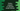# Python program to calculate simple interest# Python program to calculate the simple interest using user provided values :

Simple interest is different than the compound interest. It is calculated on the main amount of loan. This amount is also known as the principal amount.

The main formula to calculate the simple interest is the same. To calculate the interest value, we need to know the principal amount, the rate of interest and the time period. This is a beginner level program and you will learn how to read user inputs, how to do basic multiplication/division and how to print out a custom variable value.

In this tutorial, we will learn how to calculate simple interest in python. Our program will read the values from the user.

The following formula is used to calculate simple interest value :

The program will ask the user to enter all three values first. The user input values will be of the floating type. We can also use integers, but the principal amount, time and interest are unlikely to have integer type always. It will then calculate the simple interest and print out the result.

## Python program :

``````#1
P = float(input("Enter the principal amount : "))
N = float(input("Enter the number of years : "))
R = float(input("Enter the rate of interest : "))
#2
SI = (P * N * R)/100
print("Simple interest : {}".format(SI))``````

You can also find the code for the above program here.

Copy the program and run it using python-3. If you are not able to run it, drop one comment below.

### Explanation :

The commented numbers in the above program denote the step numbers below :

1. Take the user input for the principal amount, time to calculate the simple interest in years and rate of interest. We are reading the input using the input() method and then converting it to a floating point number using the float() method. input() method reads user input as a string. So, we need to convert it to float first. The principal amount is stored in the variable P, the number of years in N and the rate of interest in R.
2. Now, calculate the simple interest using the same formula we mentioned at the starting of the program. Finally, print it out. We are using {} and .format() to print out the final result.

### Extension of the program :

What you can do more with this program? If you are a beginner in programming, you can create a simple Application to calculate the simple interest. That will be a great thing to put in your resume, don’t you think?

Create three input fields and one button. The first field will be for ’n’, the second one for ‘p’ and the last one for ‘r’. If the user will tap on the button, calculate and print out the simple interest below the button.

You can create one simple web application using basic HTML-CSS components. Or if you want to do more, create one server and calculate the simple interest on the server. This will be a great starter project for learning a new language, what say?

### Conclusion :

In this tutorial, we have learned how to find out simple interest using user-provided values. Try to run the example above and drop one comment below if you have any queries.